Дана: 0

Барлыгы: 0,00

0

# Cube (exercises)

### Cube (exercises)

Edges, diagonals and faces of a cube can be identified by its vertices.

Математика

Этикеткалар

куб, face, edge, vertex, face diagonal, space diagonal, геометрия, solid geometry, exercise, ойын, математика

Байланысты экстралар

### Көріністер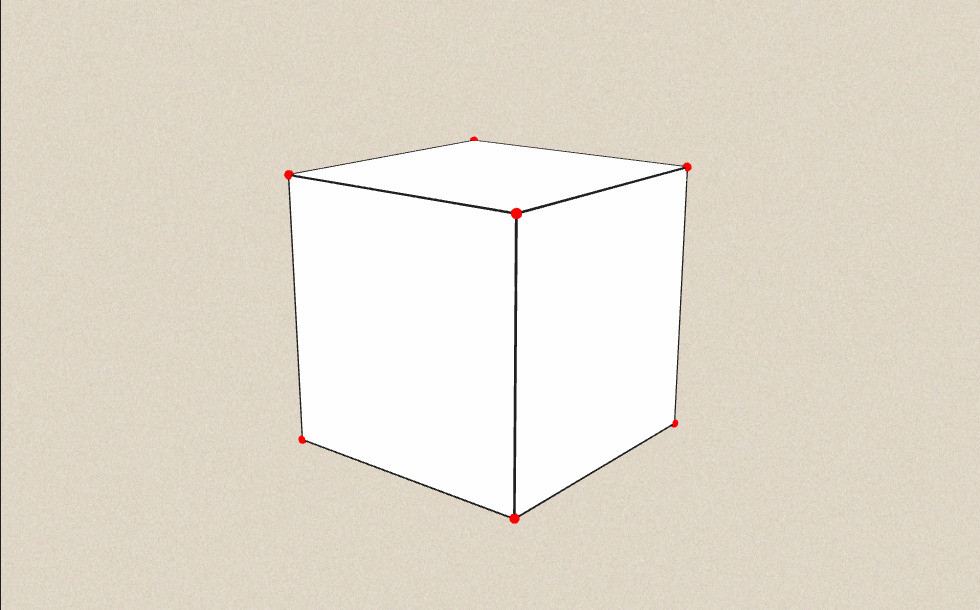### Байланысты экстралар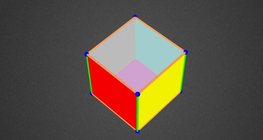#### Colouring a cube

Colouring the vertices, edges and faces of a given cube according to the criteria...#### Cube

This animation demonstrates the components (vertices, edges, diagonals and faces) of the...#### Cube of cubes

An exercise about the regular hexahedron built from unit cubes to help deepen your...#### Cube puzzle

Building cubes shown in several views from the available unit cubes aids spatial vision...#### Cube sections (exercise)

Examining solids formed by the intersection of a cube and a plane.#### Net of a cube (exercises)

Not all nets consisting of 6 congruent squares are foldable into cubes.#### Building shapes (multi colour)

Build 3D shapes from unit cubes with the help of several views.#### Building shapes (one colour)

Build 3D shapes from unit cubes with the help of several views.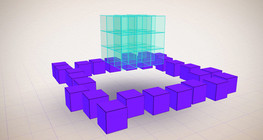#### Constructing shapes (3D)

Building 3D shapes into a given cube mesh with the help of several views.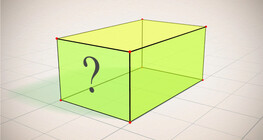#### Cuboid (exercises)

Edges, diagonals and faces of a cuboid can be identified by its vertices.#### Қысқаша көбейтудің формулалары

Анимацияда қысқаша көбейту формулаларының ұқсас қосылғыштары көрсетіледі.#### Cuboid

A cuboid is a polyhedron with six rectangular faces.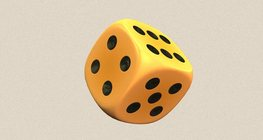#### Dice

Regular dice can be used for solving statistical and probability exercises.#### Grouping of cuboids

This animation demonstrates various types of cuboids through everyday objects.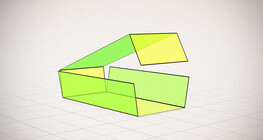#### Nets of a cuboid (exercises)

This animation demonstrates the different nets of a cuboid and includes a game.#### Volume and surface area (exercise)

An exercise about the volume and surface area of solids generated from a ´base cube´.